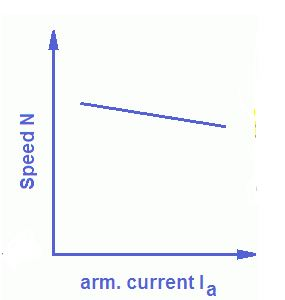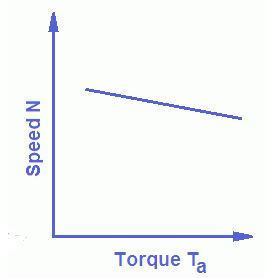# DC Shunt Motors: Characteristics & Performance

0
223

## CHARACTERISTICS OF DC SHUNT MOTOR

### TORQUE – ARMATURE CURRENT CHARACTERISTICS

We know that according to the torque equation DC motor,
T ∝  Ⲫ * I………eq 1
where, T = Torque of DC Motor.
Ⲫ = Flux produced in DC Motor.
I = Armature Current.
Since the flux Ⲫ is nearly constant in DC Shunt Motor, so eq can be written as :
T ∝  I
As we can see that from above equation that torque increases with the increase in armature current.
So, Torque-Armature Current Characteristics of DC Shunt Motor is a straight line passing through origin.Torque -Current Characteristics of DC Shunt Motor
If the effect of armature reaction is also considered, then the value of flux ⲫ decreases slightly with the increase in armature current and therefore, at higher value of Armature Current, the torque decreases slightly.

### SPEED – ARMATURE CURRENT CHARACTERISTICS

Speed of the DC Motor is given as:
N ∝ (V – I*R)/Ⲫ

And in the case of DC Shunt Motor, flux is almost constant. So the above equation can be written as :
N ∝ (V – I*R)

So from above equation it is clear that the Speed – Armature Current Characteristics of DC Shunt Motor is a straight line having a slight negative slope. That means, the speed of the motor decreases slight linearly with the increase in Armature current.Speed – Current Characteristics of  DC Shunt Motor
Since the voltage drop in armature at full load is very small as compared to applied voltage, so for all practical purposes the shunt motor is considered as constant speed motorTorque-Speed Characteristics of  DC Shunt Motor